Question

# From homework section of CD: Continuous-time convolution Consider the linear time-invariant system shown below. 7ylt) (t)...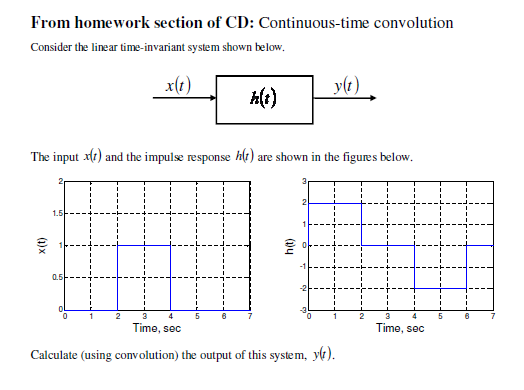From homework section of CD: Continuous-time convolution Consider the linear time-invariant system shown below. 7ylt) (t) The input alt) and the impulse response h(t) are shown in the figures below. ht) Time, sec Time, sec Calculate (using convolution) the output of this system, yo).

We need at least 10 more requests to produce the answer.

0 / 10 have requested this problem solution

The more requests, the faster the answer.

All students who have requested the answer will be notified once they are available.

#### Earn Coins

Coins can be redeemed for fabulous gifts.

Similar Homework Help Questions
• ### For a continuous time linear time-invariant system, the input-output relation is the following (x(t) the input, y(t) the...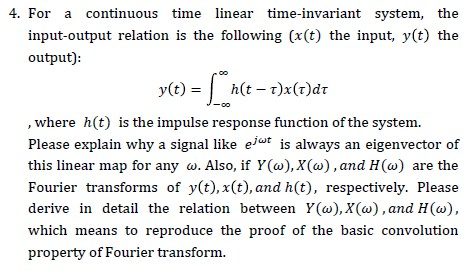For a continuous time linear time-invariant system, the input-output relation is the following (x(t) the input, y(t) the output): , where h(t) is the impulse response function of the system. Please explain why a signal like e/“* is always an eigenvector of this linear map for any w. Also, if ¥(w),X(w),and H(w) are the Fourier transforms of y(t),x(t),and h(t), respectively. Please derive in detail the relation between Y(w),X(w),and H(w), which means to reproduce the proof of the basic convolution property...

• ### Consider an linear time invariant system whose impulse response is shown in the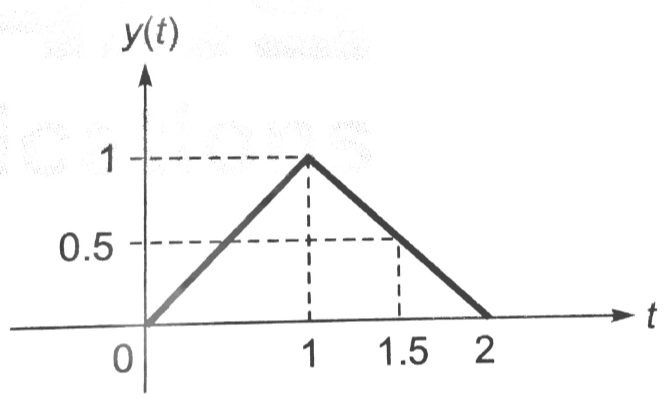Consider an linear time invariant system whose impulse response is shown in the figure below. If the input x(t) = u(t) then what will be the output at t=1.5 seconds ?

• ### 4. A linear time invariant system has the following impulse response: h(t) =2e-at u(t) Use convolution...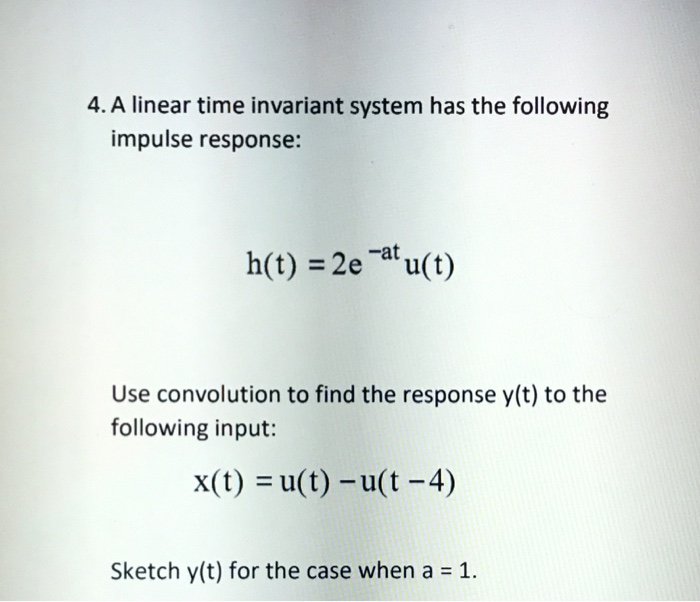4. A linear time invariant system has the following impulse response: h(t) =2e-at u(t) Use convolution to find the response y(t) to the following input: x(t) = u(t)-u(t-4) Sketch y(t) for the case when a = 1

• ### 4. Let S be a linear, time-invariant, and causal system whose input x(t) and corresponding output...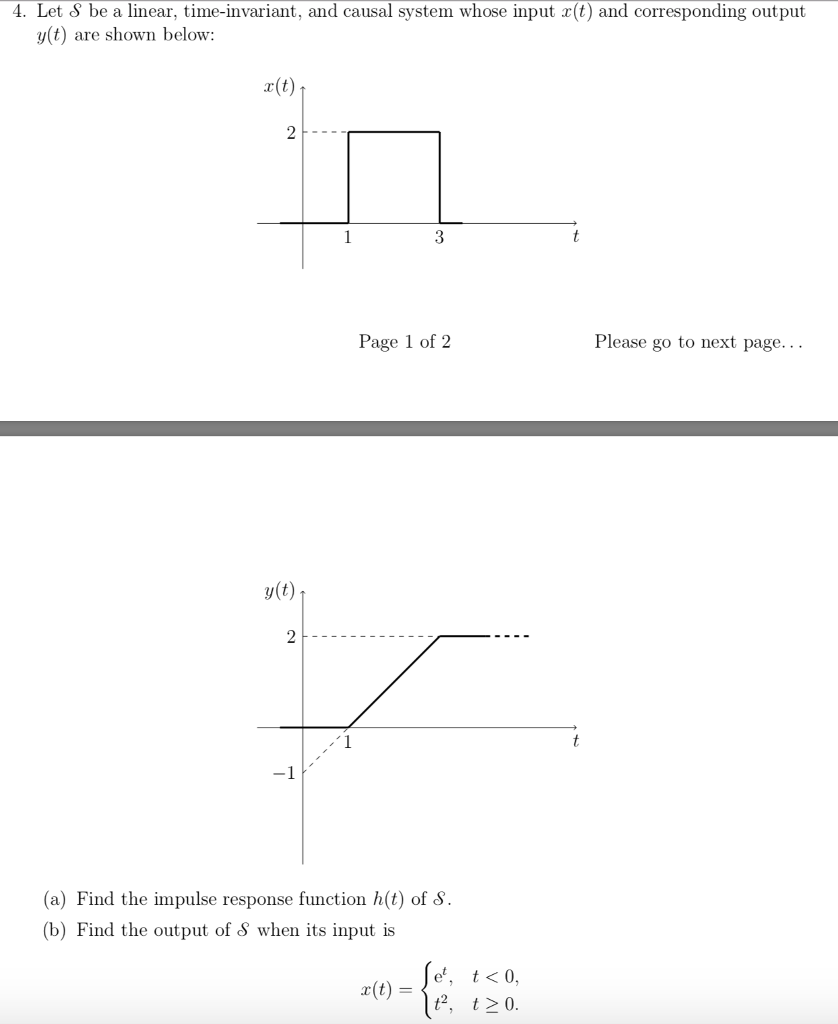4. Let S be a linear, time-invariant, and causal system whose input x(t) and corresponding output y(t) are shown below: r(t) Page 1 of 2 Please go to next page... y(t) ? (a) Find the impulse response function h(t) of ? (b) Find the output of S when its input is e*, t<0, t2, t20

• ### 2. Let y(t)(e')u(t) represent the output of a causal, linear and time-invariant continuous-time system with unit...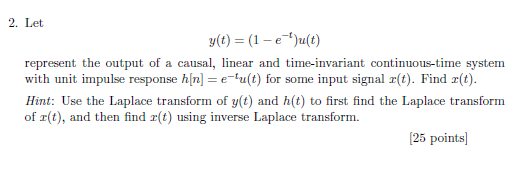2. Let y(t)(e')u(t) represent the output of a causal, linear and time-invariant continuous-time system with unit impulse response h[nu(t) for some input signal z(t). Find r(t) Hint: Use the Laplace transform of y(t) and h(t) to first find the Laplace transform of r(t), and then find r(t) using inverse Laplace transform. 25 points

• ### Determine if the linear time-invariant continuous-time system with impulse response t 1 h(t) 0. t 1...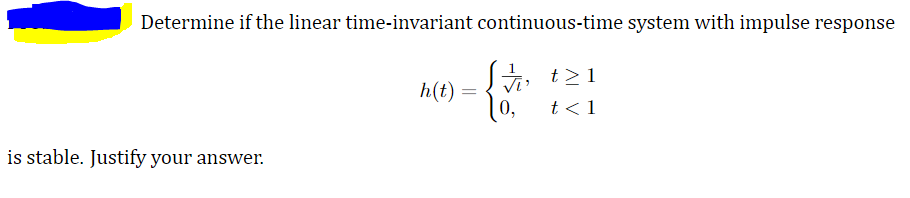Determine if the linear time-invariant continuous-time system with impulse response t 1 h(t) 0. t 1 is stable. Justify your answer

• ### Problem 4. Linear Time-Invariant System.s A linear system has the block diagram y(t) z(t) →| Delay...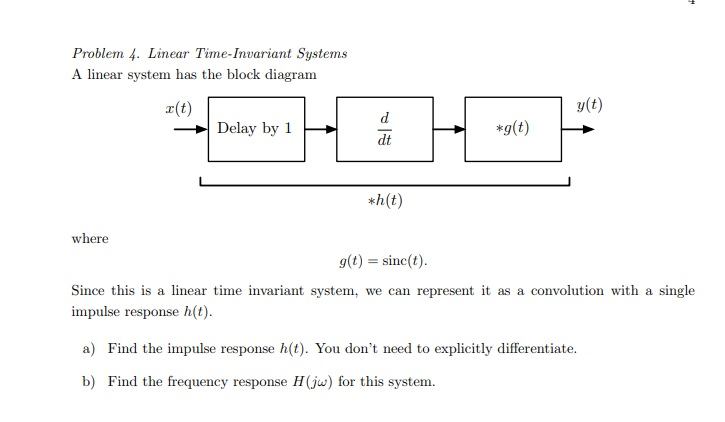Problem 4. Linear Time-Invariant System.s A linear system has the block diagram y(t) z(t) →| Delay by 1 dt *h(t) where g(t) sinc(t Since this is a linear time invariant system, we can represent it as a convolution with a single impulse response h(t) a) Find the impulse response h(t). You don't need to explicitly differentiate. b) Find the frequency response H(j for this system.

• ### 2. Consider a linear time-invariant system with transfer function H(s)Find the (s + α)(s + β) impulse response, h(t), of the system 2. Consider a linear time-invariant system with transfer f...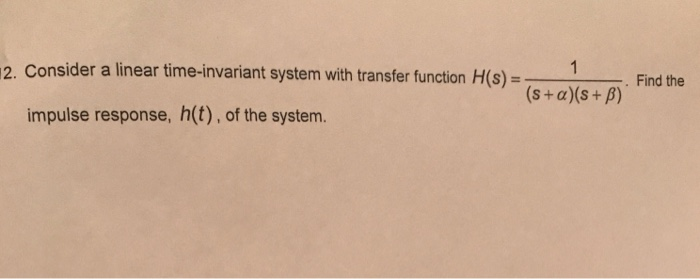2. Consider a linear time-invariant system with transfer function H(s)Find the (s + α)(s + β) impulse response, h(t), of the system 2. Consider a linear time-invariant system with transfer function H(s)Find the (s + α)(s + β) impulse response, h(t), of the system

• ### QUESTION 2 (20 MARKS) (a) A continuous time signal x(t) = 3e2tu(-t) is an input to...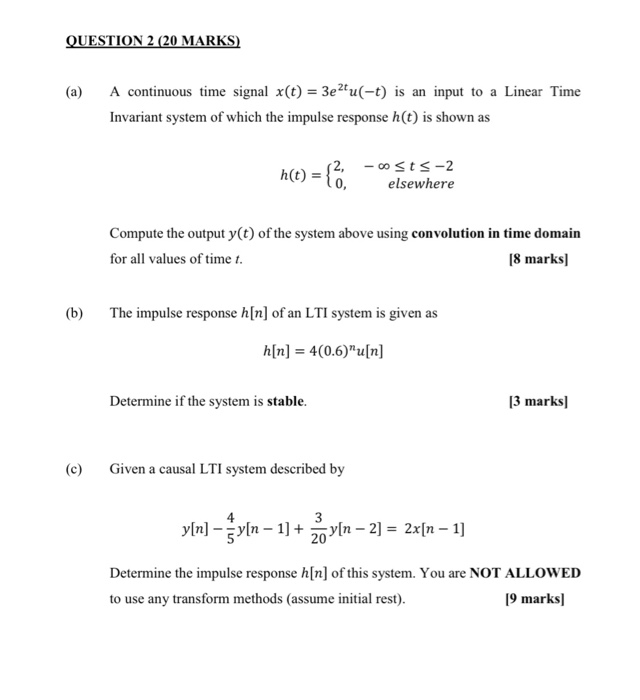QUESTION 2 (20 MARKS) (a) A continuous time signal x(t) = 3e2tu(-t) is an input to a Linear Time Invariant system of which the impulse response h(t) is shown as h(t) = { .. 12, -osts-2 elsewhere Compute the output y(t) of the system above using convolution in time domain for all values of time t. [8 marks) (b) The impulse response h[n] of an LTI system is given as a[n] = 4(0.6)”u[n] Determine if the system is stable. [3...

• ### Please dont use Laplace or Fourier A linear time-invariant continuous-time system has the impulse response h(t)...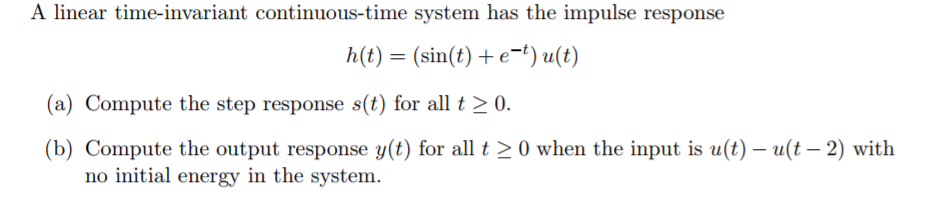Please dont use Laplace or Fourier A linear time-invariant continuous-time system has the impulse response h(t) = (sin(t) + e-t) u(t) (a) Compute the step response s(t) for all 20. (b) Compute the output response y(t) for all t > 0 when the input is u(t)-(t-2) with no initial energy in the system.